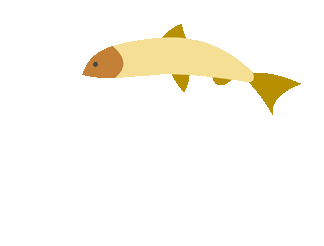top of page## Fractions & Mixed Numbers Games

##### Fraction (Resources)
###### Visual Fractions
• Visual Fractions

Identify fractions, rename fractions, compare fractions, add and subtract fractions, multiply and divide fractions, play fraction games and investigate fractions.

###### General Fraction Lessons
1. AAA Math: Fraction Lessons

Online lessons on fractions.

2. In depth notes, examples, and practice with fractions. Start with basic definitions and use the side bar to navigate your way through reducing fractions, adding and subtracting fractions and mixed numbers, multiplying and dividing fractions and mixed numbers.

3. Cool Math Lessons

Notes and examples presented in a colorful and easy to understand format. Really cool site!

4. This site is self-guided and presents like a power point that your teacher would go over with you in class.

##### Fraction (Games)
###### Visualizing Fractions
1. Visualizing Fractions

Show the given fraction on the shape.

2. Matching Models and Fractions

See what the highest level that you can get to is by matching correctly.

###### Fractions on the Number Line
1. Help Grandpa find Grandma by guessing where she is behind the hedge.

2. Find Grampy!

EasyHelp Grandma find Grandpa by guessing where she is behind the hedge.

3. Find Grampy!

It is a little harder to help Grandma find Grandpa this time, you have to be very precise and you have to be able to simplify fractions.

###### LCM and GCF
1. Greatest Common Factor

Find the greatest common factor.

2. Factors Millionaire

Play Who Wants to be a Millionaire by answering questions about factors.

3. Least Common Multiple

Find the least common multiple.

4. LCM and GCF

Find the least common multiple or greatest common factor of the given number. When you get 7 correct you get to fight the ghosts.Factors and Multiples JeopardyIdentify factors and multiples correctly and you could win jeopardy!

5. Prime Factorization, LCM, and GCF

Create factor trees for two numbers. Then, calculate the greatest common factor and least common multiple.

###### Simplifying Fractions
1. Simply Soccer

Play soccer and simplify fractions.

2. Simply Baseball

Play baseball and simplify fractions

###### Equivalent Fractions
1. Tony Fraction's Pizza Shop

Add the correct ingredients to each fraction of the pizza to make a sale. Be careful, wrong ingredients will cost you!

2. Equivalent Fractions Finder

Create equivalent fractions to match the given fraction.

3. Melvin's Make a Match

Practice matching equivalent representations of fractions and picture representations of fractions.

###### Comparing and Ordering Fractions
1. Comparing Fractions

Choose between finding the largest fraction to win the game or placing the fractions in the correct order.

2. XP Math

Compare the fractions to get the highest score before time runs out.

3. Compare fractions <,>,= that have the same denominators.

4. Compare with Un-Like Denominators

Compare fractions <,>,= that do not have the same denominators.

2. Adding and Subtracting Fractions Board Game

See who will win the game by adding and subtracting the fractions correctly and having a little bit of luck!

3. Add and Subtract Fractions with Noah

###### Adding and Subtracting with Common Denominators
1. Add and Subtract Fractions on the Number Line

Add and Subtract fractions with like denominators.

2. Add and Subtract Fractions with Fraction Circles

Add and Subtract fractions with like denominators.

###### Adding and Subtracting with Uncommon Denominators

Practice adding fractions with different denominators

2. Subtracting with Unlike Denominators

Practice subtracting fractions with different denominators

3. Word Problems: Adding and Subtracting with Unlike Denominators

Practice your problem solving skills with these fraction word problems!

###### Adding and Subtracting with Mixed Numbers
1. Adding Mixed Numbers with Unlike Denominators

Practice adding mixed numbers with different denominators.

2. Subtracting Mixed Numbers with Unlike Denominators

Practice adding mixed numbers with different denominators.

3. Word Problems: Adding and Subtracting Mixed Numbers with Unlike Denominators

Practice your problem solving skills with these mixed number word problems!

4. Study Jams
Step by Step Instructions and a 7 questions Quiz

5. Quia Quiz
Test your knowledge and compete with a partner!

###### Mixed Numbers and Improper Fractions
1. Clara Fraction's Ice Cream Shop

Convert the clients order to mixed fractions to give them the correct amount of each flavor.

2. Mixed and Improper Pac Man
Find the improper fraction that matched the mixed number shown at the bottom of the screen.

3. Quia Improper Fractions Jeopardy
Some of these may have to be simplified, for the you get them correct!

4. Fact Monster Pizzas
Slice the pizzas in the correct way, and then turn the leftovers into improper fractions, then mixed numbers.

5. XP Monster
A little bit more challenging numbers with converting improper fractions to mixed numbers.

6. IXL Math
This one only does mixed to improper fractions first, and then improper to mixed.

###### Multiplying and Dividing Fractions
1. Multiplying Fractions Soccer

Practice multiplying fractions, get the answer right and see if you can get the ball past the keeper!

2. Multiplying Fractions Millionaire

See if you are the next millionaire by correctly answering the questions correctly.

3. Dividing Fractions Soccer

Practice dividing fractions, get the answer right and see if you can get the ball past the keeper!

Practice dividing fractions, get the answer right and see if you can make the shot.

###### Dividing Mixed Numbers
1. AAA Math Dividing Mixed Numbers

Practice dividing fractions and check your work as you go so you know if you are correct or not!

###### Mixed Review Games that Have it All
1. Fractions and Mixed Number Games

Eleven different games from an easy fraction introduction to multiplying and dividing fractions.

2. Addition, Subtraction, Multiplication, and Division of Fractions Jeopardy

See if you can be the next Jeopardy Champion!

3. Fractions Booster Activity

Try out the five different fractions levels!

4. Fraction Practice

Practice your fraction skills using this interactive activity.bottom of page